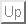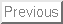Next: Introduction

# Maximum Likelihood Estimation of Logistic Regression Models: Theory and Implementation

Scott A. Czepiel1

### Abstract:

This article presents an overview of the logistic regression model for dependent variables having two or more discrete categorical levels. The maximum likelihood equations are derived from the probability distribution of the dependent variables and solved using the Newton-Raphson method for nonlinear systems of equations. Finally, a generic implementation of the algorithm is discussed.

Scott Czepiel
http://czep.net/contact.html